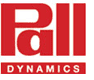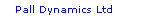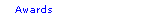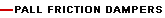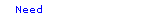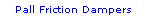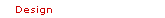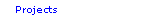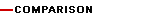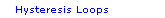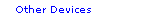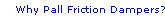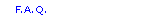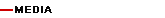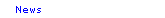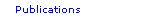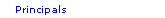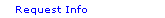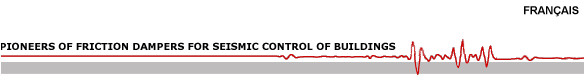Slip Load of Friction Damper The friction dampers are designed not to slip during wind. During a major earthquake, they slip prior to yielding of structural members. In general, the lower bound is about 130% of wind shear and the upper bound is 75% of the shear at which the members will yield. As seen in the diagram for Response versus Slip Load, if the slip load is very low or very high, the response is very high. Several parametric studies have shown that the slip load of the friction damper is the principal variable with the appropriate selection of which it is possible to tune the response of structure to an optimum value. Optimum slip load gives minimum response. Selection of slip load should also ensure that after an earthquake, the building returns to its near original alignment under the spring action of an elastic structure. Studies have also shown that variations up to ±25% of the optimum slip load do not affect the response significantly. Therefore, small variations in slip load (8-10%) over life of the building do not warrant any adjustments or replacement of friction damper.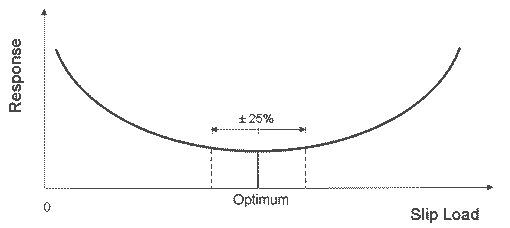Response versus Slip Load Design Criteria The quasi-static design procedure given in most building codes are ductility based and do not explicitly apply to buildings with supplemental damping. In the past few years, several guidelines on the analysis and design procedure of passive energy dissipation devices have been developed in the U.S. The latest and most comprehensive document is the "NEHRP Guidelines for the Seismic Rehabilitation of Buildings, FEMA 356 / 357, issued in 2000". The Guidelines require that the structure be evaluated for response to two levels of ground shaking - a design basis earthquake (DBE) and a maximum considered earthquake (MCE). The DBE is an event with 10% probability of exceedance in 50 years, while the MCE represents a severe ground motion of probability of 2% in 50 years. Under the DBE, the structure is evaluated to ensure that the strength demands on structural elements do not exceed their capacities and that the drift in the structure is within the acceptable limits. For the MCE, the structure is evaluated to determine the maximum displacement and overstress. It is presumed that if proper ductile detailing has been followed, the structure will have sufficient reserve to avoid collapse during MCE. Since different earthquake records, even of the same intensity, give widely varying structural responses, results obtained using a single record may not be conclusive. Therefore, three time-history records, suitable for the region should be used, one of which should be preferably site specific. The maximum response is used for the design. If seven or more earthquake records are used, the average response for design shoud be used. NEHRP guidelines require that friction dampers are designed for 130% MCE displacements and all bracing and connections are designed for 130% of damper slip load. Variation in slip load from design value should not be more that ± 15%. Non-linear Time- History Dynamic Analysis The movement of damper in an elastic brace constitutes non-linearity. Also, the amount of energy dissipation or equivalent structural damping is proportional to the displacement. Therefore, non-linear time-history dynamic analysis is a more accurate procedure for the design of buildings with damping devices. With these analyses, the time-history response of the structure during and after an earthquake can be accurately understood. The modeling of friction dampers is very simple. Since the hysteresis loop of the damper is similar to the rectangular loop of an ideal elasto-plastic material, the slip load of the friction damper can be considered as a fictitious yield force. Modeling of Pall Friction Dampers The computer modeling of Pall Friction Dampers is very easy. Since the hysteretic loop of the friction dampers is perfectly rectangular, similar to perfectly elasto-plastic material, the friction dampers can be modeled as fictitious plasticity element having yield force equal to slip load. ETABS / SAP2000, v8 Nonlinear         The single diagonal tension/compression brace with friction damper (Figure A) can be modeled as a damped brace using the following link properties:         Name = PFD1         Type = Plastic1         M = Mass of damped brace         W = Weight of damped brace         Rotational inertia 1 = Rotational inertia 2 = Rotational inertia 3 = 0         Direction = U1 (nonlinear -- put check mark in nonlinear box next to U1)                         Ke = Effective Stiffness = AE/L (properties of brace)                         Ce = Effective Damping = 0                         K = Stiffness = Ke                         Yield Strength = Slip load of friction damper                         Post Yield Stiffness Ratio = 0.0001                         Yielding exponent = 10 In this case, the brace plus friction damper (damped brace) is modeled as link element. A = Area of Brace, L = Length of Damper + Brace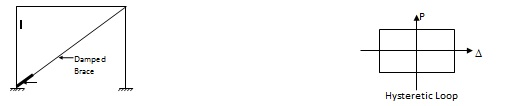Figure A: Single Diagonal Tension-Compression Brace with Pall Friction Damper The chevron friction damper (Figure B) can be modeled using the following link properties: Name = PFD1 Type = Plastic1 M = Mass of damper = 0.0013 (units: kip–inch)                                         =0.225 (units: kN-m) W = Weight of damper = 0.5 (units: kip–inch)                                         =2.22 (units: kN-m) Rotational inertia 1 = Rotational inertia 2 = Rotational inertia 3 = 0 Direction = U1 (nonlinear -- put check mark in nonlinear box next to U1)                        Ke = Effective Stiffness = 25 x damper slip load (units: kip-inch)                                                = 1000 x damper slip load (units: kN-m)                        Ce = Effective Damping = 0                        K = Stiffness = Ke                        Yield Strength = Slip load of friction damper                        Post Yield Stiffness Ratio = 0.0001                        Yielding exponent = 10 The brace is modeled as frame element. Braces are from joints A and E and joints B and E. The beams at top are from joints C and D and joints D and F. The friction damper is modeled as a nonlinear axial link element between joints D and E. Joint E can be, say, 0.01” lower and 6” away from joint D. Make sure that joint E is disconnected from the diaphragm otherwise the damper will not work or move.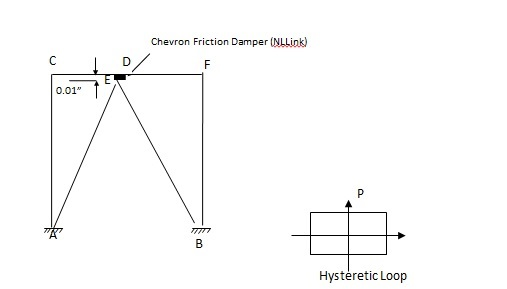Figure B: Chevron Brace with Pall Friction Damper Tension Only Cross Brace with Pall Friction Damper (Figure C) Pall Cross brace friction damper is a unique device which is suitable for long tension-only cross bracing. When tension in one of the brace forces the damper to slip, the mechanism shortens the other brace thus preventing buckling. Therefore, in the next half cycle, the other brace is ready to force the damper to slip in tension. The analysis require that each brace be modeled to slip (yield) in tension and slip in compression at almost zero load without buckling. Computer programs like DRAIN-3DX have the capability of modeling nonlinear link element with different yield values for tension and compression. Since ETABS and SAP2000 have only one value for yielding in tension and compression, the following equivalent approach is followed. The hysteretic loop of each tension brace is equal to hysteretic loop of one single diagonal tension-compression brace having half the slip load. However, the brace and the connections should be designed considering the full slip load.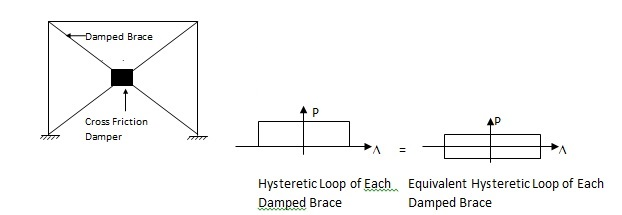Figure C: Tension Only Cross Brace with Pall Friction Damper